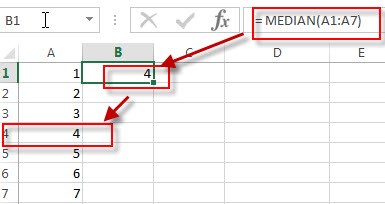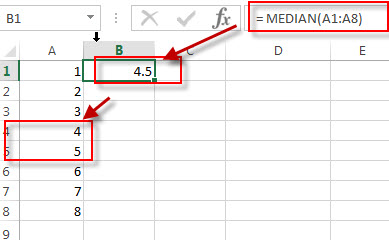# Excel MEDIAN Function

This post will guide you how to use the MEDIAN function with syntax and examples in Microsoft excel.

### Description

The Excel MEDIAN function returns the median of the given numbers. And the median is the number in the middle of a list of supplied numbers.  For example, if a list of supplied numbers are 3, 5, 6, 7, 8, the MEDIAN function will return the middle number in this list, it is number 6.

The MEDIAN function is a build-in function in Microsoft Excel and it is categorized as a Statistical Function.

The MEDIAN function is available in Excel 2016, Excel 2013, Excel 2010, Excel 2007, Excel 2003, Excel XP, Excel 2000, Excel 2011 for Mac.

### Syntax

The syntax of the MEDIAN function is as below:

= MEDIAN (number1, [number2], …)

Where the MEDIAN function arguments are:

• number1 – This is a required argument.  A number or array or cell reference that contain only numeric values.
• number2-This is an optional argument.  A number or array or reference that refers to numeric values. It can be up to 255 numeric values in Excel 2007 or later. And it only can be up to 30 number arguments in Excel 2003.

Note:

• If the total number of supplied number arguments is odd, the MEDIAN function will calculate the middle number in the list of numbers.
• If the total number of supplied number arguments is even, the MEDIAN function will calculate the average of the two numbers in the middle.
• Any arguments can either be numeric values or Defined names, arrays, or cell reference that contain only numeric values.
• If the list of number arguments contain logical values text, or empty cells, those values will be ignored.

### Excel MEDIAN Function Examples

The below examples will show you how to use Excel MEDIAN Function to get the middle number of a group of a supplied numbers.

Example 1:  to get the median of the 8 numbers in the range A1:A6. Because the list have 7 values, the fourth is the middle number, using the following formula:

=

MEDIAN (A1:A7)Example 2:  to get the median of the 8 numbers in the range A1:A8. Because the total number of supplied list is even, it will calculate the average the two numbers in the middle, using the following formula:

=

MEDIAN (A1:A8)### Related Functions

• Excel AVERAGE function
The Excel AVERAGE function returns the average of the numbers that you provided.The syntax of the AVERAGE function is as below:=AVERAGE (number1,[number2],…)….
Related Posts

How to Calculate Total Work Days or Hours Exclude Weekends/Holidays in Excel

In our daily work we often need to count the total working-days or working-hours for employees then we can pay for them the salary in some cases. But we have to count the total exclude weekends or holidays as we ...

How to Calculate the Median in Different Cases in Excel

The median is the number in the middle of a range of data by order, half of the data in this range is larger than it, and half is smaller than it. Sometimes we want to calculate the median for ...

Excel DSTDEVP Function

This post will guide you how to use Excel DSTDEVP function with syntax and examples in Microsoft excel. Description The Excel DSTDEVP returns the standard deviation of a population based on the entire population of numbers in a column or ...

Excel DSTDEV Function

This post will guide you how to use Excel DSTDEV function with syntax and examples in Microsoft excel. Description The Excel DSTDEV returns the standard deviation of a population based on a sample of numbers in a column or database ...

Excel DMIN Function

This post will guide you how to use Excel DMIN function with syntax and examples in Microsoft excel. Description The Excel DMIN returns the minimum value from a database or a column of a list that matches the specified conditions. ...

Excel DPRODUCT Function

This post will guide you how to use Excel DPRODUCT function with syntax and examples in Microsoft excel. Description The Excel DPRODUCT returns the product of values from a set of records that match criteria that you specify. The DPRODUCT ...

Excel XIRR Function

This post will guide you how to use Excel XIRR function with syntax and examples in Microsoft excel. Description The Excel XIRR function returns the internal rate of return for a series of cash flows that is not necessarily periodic. ...

Excel VDB Function

This post will guide you how to use Excel VDB function with syntax and examples in Microsoft excel. Description The Excel VDB function calculates the depreciation of an asset for a specified period based on the double declining balance method. ...

Excel SYD Function

This post will guide you how to use Excel SYD function with syntax and examples in Microsoft excel. Description The Excel SYD function calculates the sum-of-years’digits depreciation of an asset for a specified period. And it will return a numeric ...

Excel SLN Function

This post will guide you how to use Excel SLN function with syntax and examples in Microsoft excel. Description The Excel SLN function calculates the depreciation of an asset for one period based on the straight line depreciation. And it ...

Sidebar